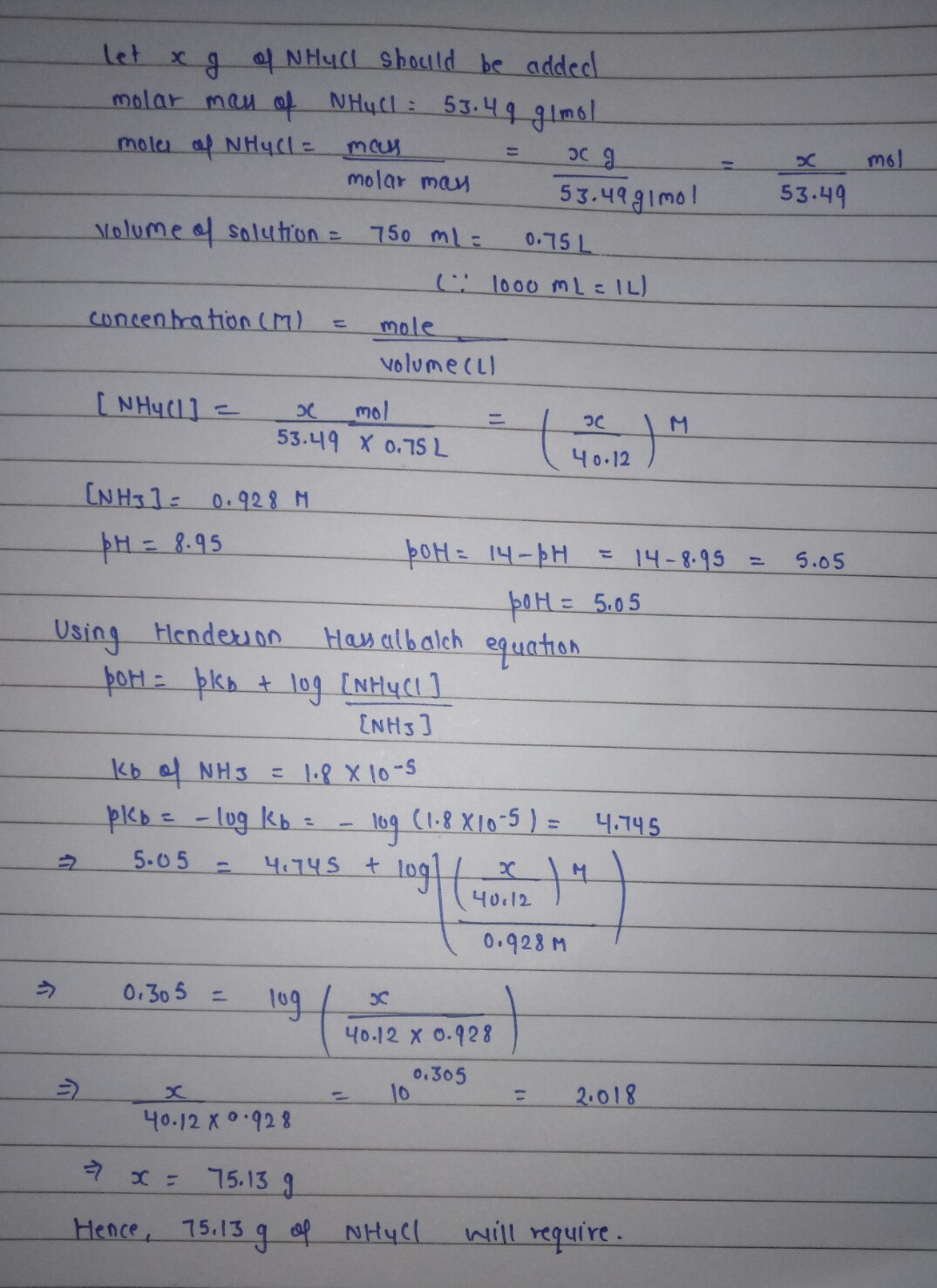Question

# How many grams (to the nearest 0.01 g) of NH4Cl (Mm = 53.49 g/mol) must be...

How many grams (to the nearest 0.01 g) of NH4Cl (Mm = 53.49 g/mol) must be added to 750. mL of 0.928-M solution of NH3 in order to prepare a pH = 8.95 buffer?#### Earn Coins

Coins can be redeemed for fabulous gifts.

Similar Homework Help Questions
• ### How many grams (to the nearest 0.01 g) of NH4Cl (Mm = 53.49 g/mol) must be...

How many grams (to the nearest 0.01 g) of NH4Cl (Mm = 53.49 g/mol) must be added to 750. mL of 1.188-M solution of NH3 in order to prepare a pH = 9.90 buffer?

• ### How many grams (to the nearest 0.01 g) of NH4Cl (Mm = 53.49 g/mol) must be...

How many grams (to the nearest 0.01 g) of NH4Cl (Mm = 53.49 g/mol) must be added to 550. mL of 0.976-M solution of NH3 in order to prepare a pH = 9.15 buffer? What volume (to the nearest 0.1 mL) of 4.00-M NaOH must be added to 0.600 L of 0.300-M HNO2 to prepare a pH = 3.50 buffer?

• ### How many grams of KCN (Mm = 65.1 g/mol) must be added to 900. mL of...

How many grams of KCN (Mm = 65.1 g/mol) must be added to 900. mL of 1.240-M solution of HCN in order to prepare a pH = 8.86 buffer?

• ### How many grams of dry NH4Cl need to be added to 1.50 L of a 0.700...

How many grams of dry NH4Cl need to be added to 1.50 L of a 0.700 M solution of ammonia, NH3, to prepare a buffer solution that has a pH of 8.56? Kb for ammonia is 1.8×10−5.

• ### How many grams of dry NH4Cl need to be added to 1.90 L of a 0.800...

How many grams of dry NH4Cl need to be added to 1.90 L of a 0.800 M solution of ammonia, NH3, to prepare a buffer solution that has a pH of 8.74? Kb for ammonia is 1.8×10^-5.

• ### How many grams of dry NH4Cl need to be added to 2.00 L of a 0.700...

How many grams of dry NH4Cl need to be added to 2.00 L of a 0.700 M solution of ammonia, NH3, to prepare a buffer solution that has a pH of 9.00? Kb for ammonia is 1.8×10−5. Express your answer with the appropriate units.

• ### How many grams of dry NH4Cl need to be added to 1.90 L of a 0.800...

How many grams of dry NH4Cl need to be added to 1.90 L of a 0.800 M solution of ammonia, NH3, to prepare a buffer solution that has a pH of 8.74? Kb for ammonia is 1.8×10^−5. Express your answer with the appropriate units.

• ### 4B. How many grams of dry NH4Cl need to be added to 1.80 L of a...

4B. How many grams of dry NH4Cl need to be added to 1.80 L of a 0.100 M solution of ammonia, NH3, to prepare a buffer solution that has a pH of 8.93? Kb for ammonia is 1.8×10^−5. Express your answer with the appropriate units.

• ### How many grams of dry NH4Cl need to be added to 2.50 L of a 0.500 M solution of ammonia, NH3, to prepare a buffer soluti...

How many grams of dry NH4Cl need to be added to 2.50 L of a 0.500 M solution of ammonia, NH3, to prepare a buffer solution that has a pH of 8.74? Kb for ammonia is 1.8*10^-5.

• ### How many grams of dry NH4Cl need to be added to 1.70 L of a 0.100 M solution of ammonia, NH3, to prepare a buffer solu...

How many grams of dry NH4Cl need to be added to 1.70 L of a 0.100 M solution of ammonia, NH3, to prepare a buffer solution that has a pH of 8.62? Kb for ammonia is 1.8×10−5.